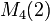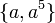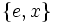# M16

View a complete list of particular groups (this is a very huge list!)[SHOW MORE]

## Definition

The group, sometimes denoted$M_{16}$ or$M_4(2)$, is defined as follows:$M_{16} = \langle a,x \mid a^8 = x^2 = e, xax^{-1} = a^5 \rangle$.

Here,$e$ denotes the identity element.

## Position in classifications

Type of classification Position/number in classification
GAP ID$(16,6)$, i.e.,$6^{th}$ among groups of order 16
Hall-Senior number 12 among groups of order 16
Hall-Senior symbol$16\Gamma_2d$

## Arithmetic functions

Want to compare and contrast arithmetic function values with other groups of the same order? Check out groups of order 16#Arithmetic functions
Function Value Similar groups Explanation for function value
underlying prime of p-group 2
order (number of elements, equivalently, cardinality or size of underlying set) 16 groups with same order
prime-base logarithm of order 4 groups with same prime-base logarithm of order
max-length of a group 4 max-length of a group equals prime-base logarithm of order for group of prime power order
chief length 4 chief length equals prime-base logarithm of order for group of prime power order
composition length 4 composition length equals prime-base logarithm of order for group of prime power order
exponent of a group 8 groups with same order and exponent of a group | groups with same exponent of a group cyclic subgroup of order 8.
prime-base logarithm of exponent 3 groups with same order and prime-base logarithm of exponent | groups with same prime-base logarithm of order and prime-base logarithm of exponent | groups with same prime-base logarithm of exponent
nilpotency class 2 groups with same order and nilpotency class | groups with same prime-base logarithm of order and nilpotency class | groups with same nilpotency class
derived length 2 groups with same order and derived length | groups with same prime-base logarithm of order and derived length | groups with same derived length the derived subgroup is contained in the cyclic subgroup and is hence abelian
Frattini length 3 groups with same order and Frattini length | groups with same prime-base logarithm of order and Frattini length | groups with same Frattini length
minimum size of generating set 2 groups with same order and minimum size of generating set | groups with same prime-base logarithm of order and minimum size of generating set | groups with same minimum size of generating set
subgroup rank of a group 2 groups with same order and subgroup rank of a group | groups with same prime-base logarithm of order and subgroup rank of a group | groups with same subgroup rank of a group All proper subgroups are cyclic, dihedral, or Klein four-groups.
rank of a p-group 2 groups with same order and rank of a p-group | groups with same prime-base logarithm of order and rank of a p-group | groups with same rank of a p-group there exist Klein four-subgroups.
normal rank of a p-group 2 groups with same order and normal rank of a p-group | groups with same prime-base logarithm of order and normal rank of a p-group | groups with same normal rank of a p-group all abelian normal subgroups are cyclic.
characteristic rank of a p-group 2 groups with same order and characteristic rank of a p-group | groups with same prime-base logarithm of order and characteristic rank of a p-group | groups with same characteristic rank of a p-group There is a unique (hence characteristic) Klein four-subgroup.

## Group properties

### Important properties

Property Satisfied? Explanation Comment
group of prime power order Yes
nilpotent group Yes prime power order implies nilpotent
supersolvable group Yes via nilpotent: finite nilpotent implies supersolvable
solvable group Yes via nilpotent: nilpotent implies solvable
abelian group No$a,x$ do not commute
metacyclic group Yes has cyclic subgroup$\langle a \rangle$ of order eight, quotient group isomorphic to$\langle x \rangle$ is cyclic of order two.
metabelian group Yes follows from being metacyclic.

### Other properties

Property Satisfied? Explanation Comment
finite group that is 1-isomorphic to an abelian group Yes via cocycle halving generalization of Baer correspondence See element structure of groups of order 16#1-isomorphism
Schur-trivial group Yes
directly indecomposable group Yes cannot be expressed as a direct product of proper subgroups; all proper subgroups are abelian, but the group is non-abelian.
splitting-simple group No it is an internal semidirect product of$\langle a \rangle$ (a normal subgroup) and$\langle x \rangle$.
centrally indecomposable group Yes it cannot be expressed as a central product of strictly smaller subgroups.
group of nilpotency class two Yes
group in which every normal subgroup is characteristic No$\langle a \rangle$ is normal but not characteristic.
ambivalent group No$a,a^{-1}$ are not conjugate. This also means that it is not a rational group or rational-representation group.

## Elements

Further information: element structure of M16

### Conjugacy class structure

Conjugacy class Size of conjugacy class Order of elements in conjugacy class Centralizer of first element of class$\{ e \}$ 1 1 whole group$\{ a^4 \}$ 1 2 whole group$\{ a^2 \}$ 1 4 whole group$\{ a^6 \}$ 1 4 whole group$\{ a, a^5 \}$ 2 8$\langle a \rangle$$\{ a^3, a^7 \}$ 2 8$\langle a \rangle$$\{ x, a^4x \}$ 2 2$\langle a^2, x \rangle$$\{ a^2x, a^6x \}$ 2 4$\langle a^2, x \rangle$$\{ ax, a^5x \}$ 2 8$\langle ax \rangle$$\{ a^3x, a^7x \}$ 2 8$\langle ax \rangle$

### Automorphism class structure

Equivalence class under automorphisms Size of equivalence class Number of conjugacy classes in it Size of each conjugacy class Characterization(s) up to 1-isomorphism Characterization(s) involving commutation relationships$\! \{ e \}$ 1 1 1 identity element identity element$\! \{ a^4 \}$ 1 1 1 unique non-identity element that is a fourth power unique non-identity element that is a commutator$\! \{ a^2, a^6 \}$ 2 2 1 squares that are not fourth powers elements in the center but not in the derived subgroup$\! \{ a, a^3, a^5, a^7, ax, a^3x, a^5x, a^7x \}$ 8 4 2 elements of order eight$\! \{ x, a^4x \}$ 2 1 2 elements of order two that are not squares$\! \{ a^2x, a^6x \}$ 2 1 2 elements of order four that are not squares

### 1-isomorphism

The group is 1-isomorphic to the group direct product of Z8 and Z2. In other words, there is a bijection between the groups that restricts to an isomorphism on all cyclic subgroups on either side. The 1-isomorphism is explained by the cocycle halving generalization of Baer correspondence, where the intermediary is a class two Lie cring.

## Subgroups

Further information: subgroup structure of M16

To describe subgroups, we use the defining presentation given at the beginning:$M_{16} = \langle a,x \mid a^8 = x^2 = e, xax = a^5 \rangle$.

Automorphism class of subgroups List of subgroups Isomorphism class Order of subgroups Index of subgroups Number of conjugacy classes(=1 iff automorph-conjugate subgroup) Size of each conjugacy class(=1 iff normal subgroup) Total number of subgroups(=1 iff characteristic subgroup) Isomorphism class of quotient (if exists) Subnormal depth Nilpotency class
trivial subgroup$\{ e \}$ trivial group 1 16 1 1 1 M16 1 0
derived subgroup of M16$\{ e, a^4 \}$ cyclic group:Z2 2 8 1 1 1 direct product of Z4 and Z2 1 1
other subgroups of order two$\{ e, x \}$,$\{ e, a^4x \}$ cyclic group:Z2 2 8 1 2 2 -- 2 1
center of M16$\{ e, a^2, a^4, a^6 \}$ cyclic group:Z4 4 4 1 1 1 Klein four-group 1 1
other cyclic subgroup of order 4$\{ e, a^2x, a^4, a^6x \}$ cyclic group:Z4 4 4 1 1 1 cyclic group:Z4 1 1
V4 in M16$\{ e, x, a^4, a^4x \}$ Klein four-group 4 4 1 1 1 cyclic group:Z4 1 1
Z8 in M16$\{ e,a,a^2,a^3,a^4,a^5,a^6,a^7 \}$$\{ e,ax,a^6,a^7x,a^4,a^5x,a^2,a^3x\}$
cyclic group:Z8 8 2 2 1 2 cyclic group:Z2 1 1
direct product of Z4 and Z2 in M16$\{ e,a^2,a^4,a^6,x,a^2x,a^4x,a^6x \}$ direct product of Z4 and Z2 8 2 1 1 1 cyclic group:Z2 1 1
whole group all elements M16 16 1 1 1 1 trivial group 1 1
Total (9 rows) -- -- -- -- 10 -- 11 -- -- --

## GAP implementation

### Group ID

This finite group has order 16 and has ID 6 among the groups of order 16 in GAP's SmallGroup library. For context, there are 14 groups of order 16. It can thus be defined using GAP's SmallGroup function as:

SmallGroup(16,6)

For instance, we can use the following assignment in GAP to create the group and name it$G$:

gap> G := SmallGroup(16,6);

Conversely, to check whether a given group$G$ is in fact the group we want, we can use GAP's IdGroup function:

IdGroup(G) = [16,6]

or just do:

IdGroup(G)

to have GAP output the group ID, that we can then compare to what we want.

### Hall-Senior number

This group of prime power order has order 16 and has Hall-Senior number 11 among the groups of order 16. This information can be used to construct the group in GAP using the Gap3CatalogueGroup function as follows:

Gap3CatalogueGroup(16,11)

WARNING: There is some disagreement between the GAP 3 catalogue numbers and the Hall-Senior numbers for some abelian groups, but it does not affect this group.

For instance, we can use the following assignment in GAP to create the group and name it$G$:

gap> G := Gap3CatalogueGroup(16,11);

Conversely, to check whether a given group$G$ is in fact the group we want, we can use GAP's Gap3CatalogueIdGroup function:

Gap3CatalogueIdGroup(G) = [16,11]

or just do:

Gap3CatalogueIdGroup(G)

to have GAP output the group ID, that we can then compare to what we want.

### Description by presentation

The group can be defined using a presentation as follows:

gap> F := FreeGroup(2);;
gap> G := F/[F.1^8,F.2^2,F.2*F.1*F.2*F.1^(-5)];
<fp group on the generators [ f1, f2 ]>
gap> IdGroup(G);
[ 16, 6 ]